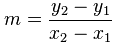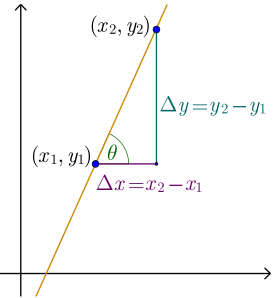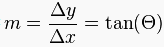### Introduction

In mathematics, the slope or gradient of a line is a number that describes both the direction and the steepness of the line.

• The direction of a line is either increasing, decreasing, horizontal or vertical.
• A line is increasing if it goes up towards the right. The slope is positive, i.e. m > 0.
• A line is decreasing if it goes down towards the right. The slope is negative, i.e. m < 0.
• If a line is horizontal the slope is zero. This is a constant function.
• If a line is vertical the slope is undefined.
• The steepness, incline, or grade of a line is measured by the absolute value of the slope. A slope with a greater absolute value indicates a steeper incline (or decline if the slope itself is negative).

Slope is calculated by finding the ratio of the "vertical change" divided by the "horizontal change" between (any) two distinct points on a line. This ratio is a number and it is the same number for every two distinct points on the same line.

In mathematical language, the slope m of the line is:The concept of slope applies directly to grades or gradient in geography. Through trigonometry, the grade m of a road is related to its angle of incline θ by m = tanθ.